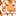# QlikView App Dev

Discussion Board for collaboration related to QlikView App Development.

Announcements
Skip the ticket, Chat with Qlik Support instead for instant assistance.
cancel
Showing results for
Did you mean:Creator

## Expression help

=If(GetSelectedCount(LuCurrencyType3) > 0,

num(sum(if([Expense Type]='Base Rent',

If([Year of Expense] = max([Year of Expense]),

[Expense Amount (USD)]/Factors))), '\$(varCurrencySymbol) #,##0'),

num(sum(if([Expense Type]='Base Rent',

If([Year of Expense] = max([Year of Expense]),

[Expense Amount (USD)]))), '\$(varCurrencySymbol) #,##0'))

Here I am getting an error saying Nested Aggregation not allowed and I guess it is because of

If([Year of Expense] = max([Year of Expense]),

How can I put this together.

1 Solution

Accepted SolutionsMVP

Maybe try

If([Year of Expense] = max(TOTAL [Year of Expense]),

or specify what exactely you want to achieve and how your model & data looks like.

3 RepliesMVP

Maybe try

If([Year of Expense] = max(TOTAL [Year of Expense]),

or specify what exactely you want to achieve and how your model & data looks like.MVP

You need to add an aggr() function after your sum because of what you are trying to do.... Alternatively, may be you just need to use set analysis if your comparison of Year of Expense to Max(Year of Expense) doesn't change based on your dimensions?Creator
Author

Thnak you very much.That helped.Community Browser